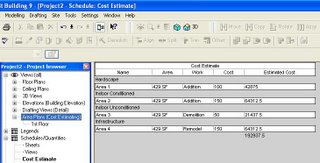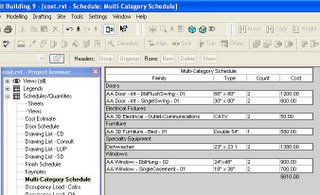## Wednesday, August 23, 2006

### Cost Estimating

There are 3 ways we've found to estimate the cost of a project in Revit.

If you want to estimate the total cost of the project per square foot then setup an Area Plan scheme and Area schedule:

1. Goto Settings... Room and Area Settings... Area Schemes... New and add a scheme called Cost Estimating.
2. Goto View... New... Area Plan... Type... Cost Estimating... and break the project down into areas of differing cost per square foot.
3. To estimate the total cost goto View... New... Schedule/Quantities... Areas (Cost Estimating) and start adding the parameters you need to estimate the total cost.
4. You'll have to add a Calculated Value called "Estimated Cost" with a formula that looks something like (Area * Cost / 1 SF) so you can total the cost of each area.If you want to estimate the total cost of the project by materials used then setup one of Revit's new Material Takeoff Schedules:

1. Some materials are estimated in Cost Per Linear Foot, some in Cost Per Square Foot, some in Cost Per Cubic Yard so I would first setup new project parameters for each estimate (Cost PLF & Cost PSF).
2. To calculate the total cost of materials goto View... New... Material Takeoff... Walls and start adding the parameters you need to calculate the cost of materials used in the walls.
3. You'll have to add a Calculated Value called "Estimated Area Cost" with a formula that looks something like (Area * Material: Cost PSF / 1 SF) so you can total the cost of material measured by area... (Length * Material: Cost PLF / 1') for "Estimated Linear Cost."
4. Now you can type in the cost for each material in the schedule. If you goto Setting... Materials... Identity you can also add a cost to each material here.

If you want to estimate the total cost of the project by unit cost then setup a Multi-Category Schedule:

1. To calculate the total cost of the project (by unit cost) goto View... New... Schedule/Quantities... Multi-Category and start adding the parameters you need to calculate the cost of all elements used. If you goto the Type Properties of each door, window, wall type, etc. you can also set a cost per unit here.
2. Now you can type in the cost for each material in the schedule.
3. Do not use Material Takeoff Schedule for estimating the total unit cost of doors and windows. Only use Material Takeoff Schedules when you want to schedule every material that is used in the door/window family separately.If you want to do cost estimating outside of Revit you might try exporting the Revit model as an ODBC and importing the database into Timberline. Haven't tried it yet myself.

David Kalimah said...

can you insert walls in the multi-category schedule?
i've been trying with no success...

Ian Darnell said...

I'm trying to make my Materials Legend act like yours. The problem is that when I enter a cost in the "Cost PLF" field, the legend updates that cost for every material in the wall type. Can you help?

i know this is a really old post, but I was wondering how you got Revit to calculate the length of a material. For the life of me it won't me multiply the Cost PLF parameter by the length.

Rob Cross said...

Hi there,

"4. Now you can type in the cost for each material in the schedule. If you go to Setting... Materials... Identity you can also add a cost to each material here."

Can this be done on Revit Linked files?